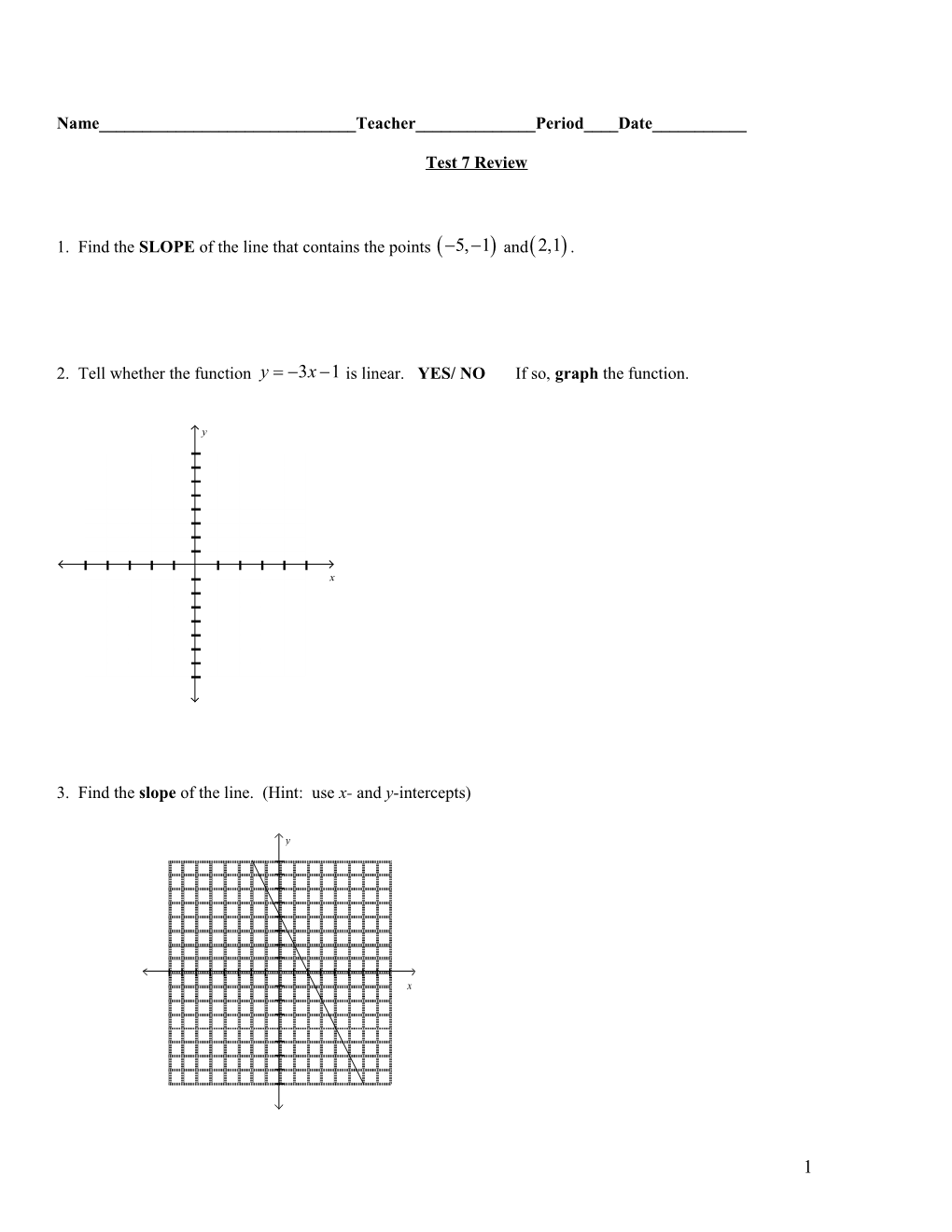# Test Chapter 5 and Chapter 6 Section 5Name______Teacher______Period____Date______

Test 7 Review

1. Find the SLOPE of the line that contains the points and.

2. Tell whether the function is linear. YES/ NO If so, graph the function.

3. Find the slope of the line. (Hint: use x- and y-intercepts)

4. Determine if the relationship in the table is linear.

If it is linear write the equation of the line in slope-intercept form.

x / y
0 / -3
2 / -6
4 / -9
6 / -12

Is it Linear? Circle Yes or No

If yes, the equation of the line is y = ______

# of Hours /

2 / \$25.00
4 / \$50.00
6 / \$75.00

## 6. Describe in words what the rate of change means in Kirk’s scenario.

7. Tell whether (5, 8) is a solution of .YES/NO

8. Write the equation of the line perpendicular to that goes through the point.

9. Gracie invited two hundred people to her

15th birthday party. The banquet hall charges

\$27.50 per person plus \$200 for the DJ. The

total charge can be expressed as a linear

function of people attending. What is the slope

of this linear function?

m = ______

Be sure to use the correct place value.

10. Graph the solutions of the linear inequality . (Hint: solve for y first)1. Write an inequality to represent the graph. (Hint: the line is dashed).

12. GRAPHand. Then DESCRIBE THE TRANSFORMATION from the graph of TO the graph of. GRAPH BOTH LINES AND CHOOSE ONE ANSWER FROM A AND B.

1. g(x) is translated up/down from f(x)
1. g(x) is less steep/steeper than f(x)

13. Write an equation in slope-intercept form of the line with slope that contains the point (3, –7).

14. The graph represents the cost of buying containers for a fundraiser. If 75 containers are purchased, which company offers the best price?

15. The graph shows a linear relationship. Find the SLOPE. (Hint: use the x- and y-intercepts)

16. Find the x- and y-intercepts of .

17. Tell whether the SLOPE of the line is positive, negative, zero, or undefined.

18. If y= 2.50x + 5 is a function relating the cost y of riding x number of rides at a carnival, what does the slope represent?

19. Find the x- and y-intercepts.

20. Graph the linear function and its transformation. Describe the transformation from f(x) tog(x).

21. A music club charges an initial joining fee of \$15.00. The cost per CD is \$12.00. Write the equation that represents this situation in terms of x and y. What about the equation will change if the cost per CD goes upby \$2.00? Write the new equation in terms of x and y.

Original equation______

New equation______

1